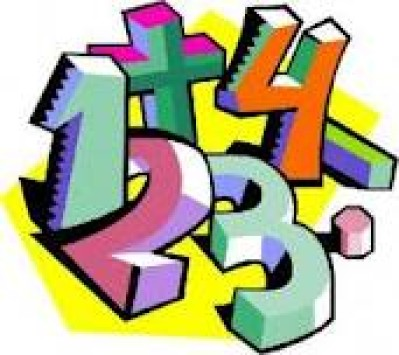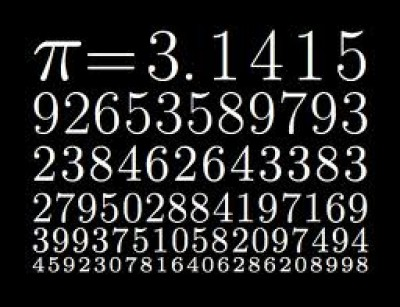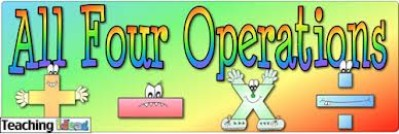List of public pages created with Protopage

# Home

## Plain sticky notes

### Decimals

Decimals are a method of writing fractional numbers without writing a fraction having a numerator and denominator. The fraction 7/10 could be written as the decimal 0.7. The period or decimal point indicates that this is a decimal. Or you can have these? 3/10=0.3 1/2=0.5 1/4=0.25 4/5=0.8 2/5=0.4 1/6=0.16

### Fractions

Fractions consist of two numbers. The top number is called the numerator. The bottom number is called the denominator. For example... To add two fractions with the same denominator, add the numerators and place that sum over the common denominator. 4/5+3/5=9/5. 3/9+4/9=7/9

### Percentages

In mathematics, a percentage is a number or ratio as a fraction of 100. For example...45% is equal to 45/100 and 0.45. Although percentages are usually used to express numbers between zero and one, any ratio can be expressed as a percentage. For instance, 111% is 1.11 and −0.35% is −0.0035. Although this is technically inaccurate as per the definition of percent, an alternative wording in terms of a change in an observed value is “an increase/decrease by a factor of...””

### indices

3³ ('3 cubed' or '3 to the power of 3') and 5² ('5 squared' or 5 'to the power' of 2) are example of numbers in index form. 3³ = 3×3×3 2¹ = 2 2² = 2×2 2³ = 2×2×2 etc.

## Calendars

### Calendar

• Thu October 4 - Lunchtime: Buy a new beanie hat
• Fri October 5 - 6pm: Go rollerblading

## Photos

### Maths!# Bookmarks

## Plain sticky notes

### Sticky note

Click 'edit' on the Bookmarks widget to add all of your favorite sites to your page for quick access

# Notes

## Plain sticky notes

### Sticky note

Click this text to change it...